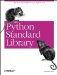# The regex Module

(Obsolete) The regex module, shown in Example 14-32, is the old (pre-1.5) regular expression machinery. New code should use re where possible.

Note that regex is faster than the re module used in Python 1.5.2, but slower than the new version used in 1.6 and later.

##### Example 14-32. Using the regex Module
```File: regex-example-1.py

import regex

text = "Mans crisis of identity in the latter half of the 20th century"

p = regex.compile("latter") # literal
print p.match(text)
print p.search(text), repr(p.group(0))

p = regex.compile("[0-9]+") # number
print p.search(text), repr(p.group(0))

p = regex.compile("") # two-letter word
print p.search(text), repr(p.group(0))

p = regex.compile("w+\$") # word at the end
print p.search(text), repr(p.group(0))

-1
32 latter
51 20
13 of
56 century```Python Standard Library (Nutshell Handbooks) with
ISBN: 0596000960
EAN: 2147483647
Year: 2000
Pages: 252
Authors: Fredrik Lundh